#load libraries:
library(strm)
library(rmarkdown)
#load package dependencies for the vignette
library(tidyr)
library(gt)
library(dplyr)
library(ggplot2)
library(patchwork)
library(purrr)
library(rgdal)
library(spdep)


strm is an R package that fits spatio-temporal regression model based on Chi & Zhu Spatial Regression Models for the Social Sciences (2019). The approach here fits a spatial error model while incorporating a temporally lagged response variable and temporally lagged explanatory variables.

This package builds on the errorsarlm() function from the spatialreg package.

This package is still under development. Please report bugs or constructive tips to issues here.

# Introduction

This data example will work through downloading census data using the tidycensus package, creating spatial weights, and then using strm to fit the spatio-temporal regression model. We will fit the same model using both the long and wide data formats.

In this example, we want to look at percent poverty as it relates to female employment rates at the Wisconsin county-level. We will use the American Community Survey (ACS) 5-year data. The variables we will extract are:

• B17020_002 - Estimate: Total - Income in the past 12 months below poverty level
• B17020_001 - Estimate: Total - Poverty Status in the past 12 months.
• B23022_026 - Estimate: Total Female by Work Status by weeks worked in the past 12 months for the population 16-64 years old.
• B23022_001 - Estimate: Total: status in the past 12 months by usual hours worked per week in the past 12 months by weeks worked in the past 12 months for the population 16-64 years old (Male and Female)

We first load in the dataset wi_raw that contains the raw 5-year estimates from two years: 2013-2017 and 2014-2018 ACS data at the county-level in Wisconsin using data(wi_raw):

data(wi_raw)


We first explore the raw Wisconsin data:

class(wi_raw)

##  "sf"         "data.frame"

names(wi_raw)

##  "GEOID"    "NAME"     "variable" "estimate" "moe"      "id"       "geometry"

str(wi_raw)

## Classes 'sf' and 'data.frame':   576 obs. of  7 variables:
##  $GEOID : chr "55043" "55043" "55043" "55043" ... ##$ NAME    : chr  "Grant County, Wisconsin" "Grant County, Wisconsin" "Grant County, Wisconsin" "Grant County, Wisconsin" ...
##  $variable: chr "B17020_001" "B17020_002" "B23022_001" "B23022_026" ... ##$ estimate: num  47928 7323 33826 15433 15929 ...
##  $moe : num 311 554 87 74 113 322 56 43 38 166 ... ##$ id      : num  2017 2017 2017 2017 2017 ...
##  $geometry:sfc_MULTIPOLYGON of length 576; first list element: List of 1 ## ..$ :List of 1
##   .. ..$: num [1:423, 1:2] -91.2 -91.2 -91.1 -91.1 -91.1 ... ## ..- attr(*, "class")= chr [1:3] "XY" "MULTIPOLYGON" "sfg" ## - attr(*, "sf_column")= chr "geometry" ## - attr(*, "agr")= Factor w/ 3 levels "constant","aggregate",..: NA NA NA NA NA NA ## ..- attr(*, "names")= chr [1:6] "GEOID" "NAME" "variable" "estimate" ...  head(wi_raw)  ## Simple feature collection with 6 features and 6 fields ## Geometry type: MULTIPOLYGON ## Dimension: XY ## Bounding box: xmin: -91.5518 ymin: 42.50706 xmax: -90.4258 ymax: 46.15791 ## Geodetic CRS: NAD83 ## GEOID NAME variable estimate moe id ## 1 55043 Grant County, Wisconsin B17020_001 47928 311 2017 ## 2 55043 Grant County, Wisconsin B17020_002 7323 554 2017 ## 3 55043 Grant County, Wisconsin B23022_001 33826 87 2017 ## 4 55043 Grant County, Wisconsin B23022_026 15433 74 2017 ## 5 55113 Sawyer County, Wisconsin B17020_001 15929 113 2017 ## 6 55113 Sawyer County, Wisconsin B17020_002 2825 322 2017 ## geometry ## 1 MULTIPOLYGON (((-91.15681 4... ## 2 MULTIPOLYGON (((-91.15681 4... ## 3 MULTIPOLYGON (((-91.15681 4... ## 4 MULTIPOLYGON (((-91.15681 4... ## 5 MULTIPOLYGON (((-91.55095 4... ## 6 MULTIPOLYGON (((-91.55095 4...  Notice that wi_raw is of class sf and class data.frame ## Long-Form Data ### Exploratory (Spatial) Data Analysis We first clean the data in order to calculate percent of each year-county population that is in poverty and percent female employment rate. We also take the natural log transformation of the poverty percent rate. We first use select() from dplyr to select out the moe (margin of error) variable as we do not need it for this analysis. We then use spread from tidyr to reformat the key variables from long to wide format so that we can then use mutate() from dplyr to create new variables (pov_prct, feemp_prct, id, logpov). Note this dataset is still in long format because there are multiple observations for each county due to multiple years. wi <- wi_raw %>% dplyr::select(-moe) %>% spread(key = variable, value=estimate) %>% mutate(pov_prct = B17020_002/B17020_001, feemp_prct = B23022_026/B23022_001, year=id, logpov = log(pov_prct)) st_crs(wi) = 4326 class(wi)  ##  "sf" "data.frame"  str(wi)  ## Classes 'sf' and 'data.frame': 144 obs. of 12 variables: ##$ GEOID     : chr  "55001" "55001" "55003" "55003" ...
##  $NAME : chr "Adams County, Wisconsin" "Adams County, Wisconsin" "Ashland County, Wisconsin" "Ashland County, Wisconsin" ... ##$ id        : num  2017 2018 2017 2018 2017 ...
##  $B17020_001: num 18962 18940 15164 15088 44510 ... ##$ B17020_002: num  2457 2794 2215 2161 5138 ...
##  $B23022_001: num 11964 11851 9889 9771 27406 ... ##$ B23022_026: num  5303 5233 4899 4798 13333 ...
##  $geometry :sfc_MULTIPOLYGON of length 144; first list element: List of 1 ## ..$ :List of 1
##   .. ..$: num [1:312, 1:2] -90 -90 -90 -90 -90 ... ## ..- attr(*, "class")= chr [1:3] "XY" "MULTIPOLYGON" "sfg" ##$ pov_prct  : num  0.13 0.148 0.146 0.143 0.115 ...
##  $feemp_prct: num 0.443 0.442 0.495 0.491 0.486 ... ##$ year      : num  2017 2018 2017 2018 2017 ...
##  $logpov : num -2.04 -1.91 -1.92 -1.94 -2.16 ... ## - attr(*, "sf_column")= chr "geometry" ## - attr(*, "agr")= Factor w/ 3 levels "constant","aggregate",..: NA NA NA NA NA NA NA NA NA NA ... ## ..- attr(*, "names")= chr [1:11] "GEOID" "NAME" "id" "B17020_001" ...  Let us explore the summary statistics by year for pov_prct using summary() and the tapply() function: summary(wi$pov_prct)

##    Min. 1st Qu.  Median    Mean 3rd Qu.    Max.
## 0.04454 0.09203 0.11502 0.11734 0.13586 0.35843

tapply(wi$pov_prct, wi$year, summary)

## $2017 ## Min. 1st Qu. Median Mean 3rd Qu. Max. ## 0.04760 0.09203 0.11505 0.11946 0.13736 0.35843 ## ##$2018
##    Min. 1st Qu.  Median    Mean 3rd Qu.    Max.
## 0.04454 0.09300 0.11475 0.11521 0.13356 0.32647


We also the summary statistics by year for feemp_prct

summary(wi$feemp_prct)  ## Min. 1st Qu. Median Mean 3rd Qu. Max. ## 0.4416 0.4841 0.4900 0.4874 0.4955 0.5122  tapply(wi$feemp_prct, wi$year, summary)  ##$2017
##    Min. 1st Qu.  Median    Mean 3rd Qu.    Max.
##  0.4432  0.4840  0.4892  0.4874  0.4954  0.5117
##
## $2018 ## Min. 1st Qu. Median Mean 3rd Qu. Max. ## 0.4416 0.4844 0.4906 0.4875 0.4958 0.5122  Finally, we look at correlation between percent poverty and percent female employment across all 2 years of ACS data: cor(wi$pov_prct,wi$feemp_prct)  ##  -0.06646265  We next visually explore the data using the geom_histogram() and geom_density() geometries from the ggplot2 package. We first explore percent poverty (pov_prct) and the log-transformed percent poverty (logpov): ggplot(data=wi, aes(x=pov_prct)) + facet_grid(.~ year) + geom_histogram(binwidth=0.01,color="black", aes(fill=factor(year))) + geom_density(alpha=.2, aes(fill=factor(year))) + theme_minimal() + xlab("Percent Poverty") + labs(title="County-Level Percent Poverty in Wisconsin Across Time", fill="Year") + theme(plot.title = element_text(hjust = 0.5), legend.position = "none") + scale_fill_manual(values=c("chartreuse4", "deeppink4", "cornflowerblue","orange2","tomato3"))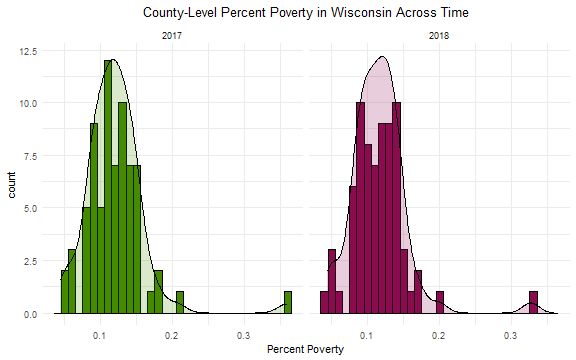breaks <- quantile(wi$logpov,seq(0,1,by=0.1))
ggplot(data=wi, aes(x=logpov)) +
geom_histogram(aes(y =..density.., fill=factor(year)),color="black", breaks=breaks) +
facet_grid(.~ year) +
geom_density(alpha=.2, aes(fill=factor(year))) +
theme_minimal() +
xlab("(Log) Percent Poverty") +
labs(title="County-Level (Log) Percent Poverty in Wisconsin Across Time", fill="Year") +
theme(plot.title = element_text(hjust = 0.5),
legend.position = "none") +
scale_fill_manual(values=c("chartreuse4", "deeppink4", "cornflowerblue","orange2","tomato3"))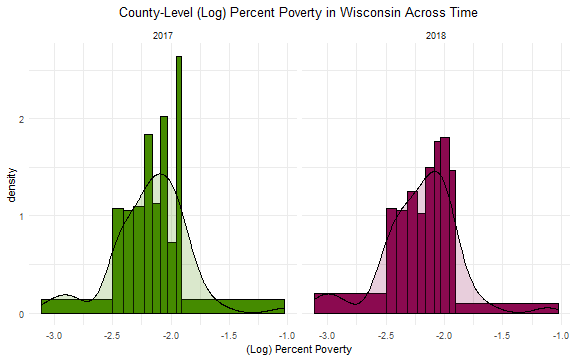Recall that wi is of class sf. We can use the geom_sf() geometry to easily create maps of both pov_prct and logpov:

ggplot(wi) +
geom_sf(aes(fill = pov_prct)) +
scale_fill_viridis_c() +
coord_sf(datum = NA) +
facet_grid(. ~ year) +
theme_minimal()+
theme(plot.title=element_text(hjust = 0.5)) +
labs(title = "County-Level Percent Poverty in Wisconsin Across Time", fill="%Poverty")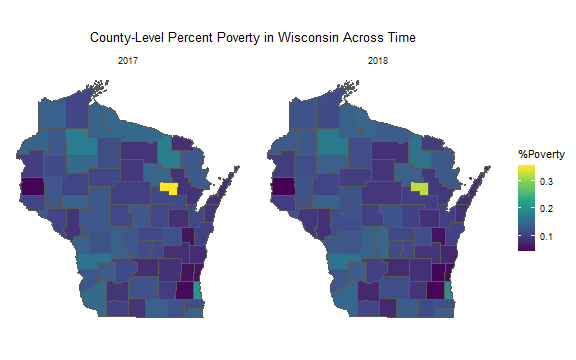ggplot(wi) +
geom_sf(aes(fill = logpov)) +
scale_fill_viridis_c() +
coord_sf(datum = NA) +
facet_grid(. ~ year) +
theme_minimal()+
theme(plot.title=element_text(hjust = 0.5)) +
labs(title = "County-Level (Log) Percent Poverty in Wisconsin Across Time", fill="(Log) %Poverty")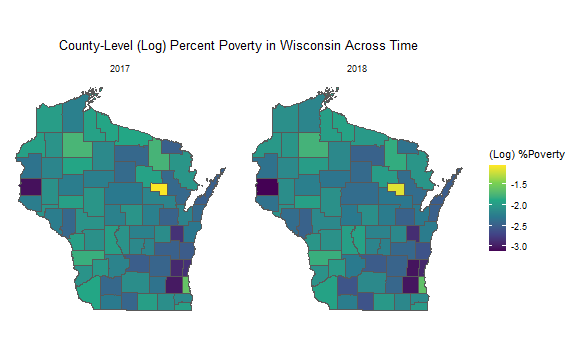Next we visually explore feemp_prct:

ggplot(data=wi, aes(x=feemp_prct)) +
facet_grid(.~ year) +
geom_histogram(binwidth=0.01,color="black", aes(fill=factor(year))) +
geom_density(alpha=.2, aes(fill=factor(year))) +
theme_minimal() +
xlab("Percent Female Employment") +
labs(title="County-Level Percent Female Employment in Wisconsin Across Time", fill="Year") +
theme(plot.title = element_text(hjust = 0.5),
legend.position = "none") +
scale_fill_manual(values=c("chartreuse4", "deeppink4", "cornflowerblue","orange2","tomato3"))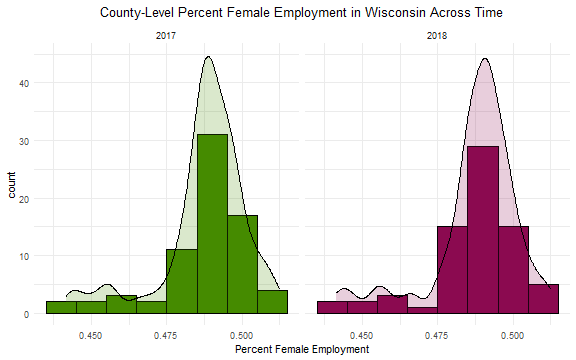ggplot(wi) +
geom_sf(aes(fill = feemp_prct)) +
scale_fill_viridis_c() +
coord_sf(datum = NA) +
facet_grid(. ~ year) +
theme_minimal()+
theme(plot.title=element_text(hjust = 0.5)) +
labs(title = "County-Level Percent Female Employment in Wisconsin Across Time", fill="%Feemp")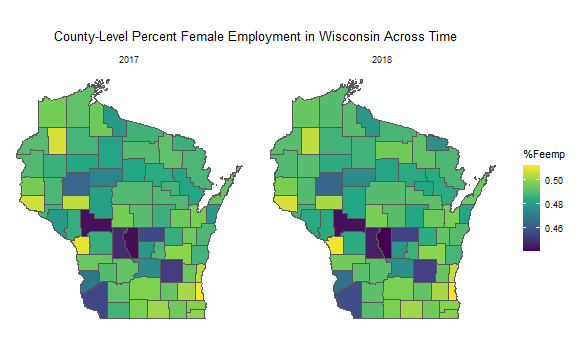### Create Neighbors

In order to apply the spatio-temporal model using strm, we need to first define county neighbors. We will define first-order contiguity-based neighborhood structure (Queen's adjacency) with row-standardized spatial weights.

We have 2 years of data, however the spatial dependence between counties remains the same. Therefore, we will select unique counties to create neighbors and spatial weights. Specifically, we will use the geometries from 2018 using filter() from dplyr and to simplify the data frame we will select just the GEOID and NAME variables using select() from dplyr:

wi_uniq <- wi %>%
dplyr::filter(year==2018) %>%
dplyr::select(GEOID, NAME)
nrow(wi_uniq)

##  72

class(wi_uniq)

##  "sf"         "data.frame"


We now have 72 unique county geometries which correspond to the 72 counties in Wisconsin.

We next create the neighborhood structure using poly2nb() from the spdep package. We set the row names of wi_uniq dataframe to correspond to the county names and then use the argument row.names=row.names(wi_uniq) in poly2nb() so that we can easily identify each element of the neighborhood structure list with a county. Finally, we print the first 6 neighbors using str(head(wi_nb)):

row.names(wi_uniq) <- wi_uniq$NAME head(row.names(wi_uniq))  ##  "Adams County, Wisconsin" "Ashland County, Wisconsin" ##  "Barron County, Wisconsin" "Bayfield County, Wisconsin" ##  "Brown County, Wisconsin" "Buffalo County, Wisconsin"  wi_nb <- poly2nb(wi_uniq, row.names=row.names(wi_uniq)) str(head(wi_nb))  ## List of 6 ##$ : int [1:6] 11 29 39 50 70 72
##  $: int [1:4] 4 26 51 58 ##$ : int [1:8] 7 9 17 49 55 56 58 66
##  $: int [1:4] 2 16 58 66 ##$ : int [1:6] 8 31 36 43 45 59
##  $: int [1:3] 18 47 62  Now that we have the neighborhood structure, we create the list of spatial weights using nb2listw() from the spdep package and print the class and first 6 elements of the spatial weights list: listw_w <- nb2listw(wi_nb, style = "W") class(listw_w)  ##  "listw" "nb"  str(head(listw_w$weights))

## List of 6
##  $: num [1:6] 0.167 0.167 0.167 0.167 0.167 ... ##$ : num [1:4] 0.25 0.25 0.25 0.25
##  $: num [1:8] 0.125 0.125 0.125 0.125 0.125 0.125 0.125 0.125 ##$ : num [1:4] 0.25 0.25 0.25 0.25
##  $: num [1:6] 0.167 0.167 0.167 0.167 0.167 ... ##$ : num [1:3] 0.333 0.333 0.333


Lastly, we can visualize these neighbors. We first extract the county polygons using st_geometry() from the sf package and save that as the object county_geoms. Next we find the county centroids using st_centroid() from sf on county_geoms and save that as the object cntrd. Lastly, we find the coordinates of the centroids using st_coordinates() on the cntrd object:

county_geoms <- st_geometry(wi_uniq)
cntrd <- st_centroid(county_geoms)
coords <- st_coordinates(cntrd)

##           X        Y
## 1 -89.77039 43.96953
## 2 -90.67794 46.31608
## 3 -91.84831 45.42368
## 4 -91.20079 46.52376
## 5 -88.00373 44.45294
## 6 -91.75445 44.37983


We can visualize the county geometries, centroids, and neighborhood:

plot(county_geoms)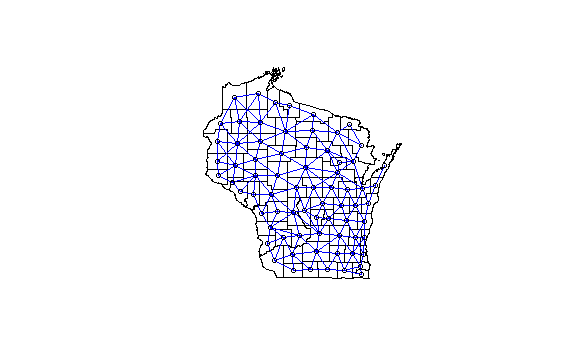### Using strm

We use the strm() function from the strm package. The first argument is the model formula. Given that the data is in long format, strm will create the lagged values for you as well as the lagged response in the right-hand side of the model. The next argument is id, which we set to “NAME” as each observation is taken at the county-level. We then specify the name of the data set (data=wi). We set the argument listw=listw_w to the row-standardized list of spatial weights. We set time=2 because there are 2 time periods in the dataset. We set wide=FALSE, thereby specifying that the data is in long format. Lastly, we set the argument returndf=TRUE to return the modified dataframe along with the model output. The default to returndf is FALSE and strm will only return the model output summary.

#create model formula
formula <-as.formula(logpov  ~ feemp_prct)
#use strm
out <- strm(formula, id="NAME", data=wi, listw = listw_w, time=2,wide=FALSE, returndf = TRUE)

## The spatio-temporal regression model fitted:

## logpov ~ feemp_prct + feemp_prct.Tlag1 + logpov.Tlag1

#explore model summary output
summary(out$res)  ## ## Call:spatialreg::errorsarlm(formula = modframe, listw = listw) ## ## Residuals: ## Min 1Q Median 3Q Max ## -0.1164060 -0.0271689 -0.0052208 0.0303804 0.1135471 ## ## Type: error ## Coefficients: (asymptotic standard errors) ## Estimate Std. Error z value Pr(>|z|) ## (Intercept) 0.490041 0.194409 2.5207 0.0117131 ## feemp_prct -9.122181 2.765473 -3.2986 0.0009717 ## feemp_prct.Tlag1 8.009282 2.792433 2.8682 0.0041280 ## logpov.Tlag1 0.992349 0.018244 54.3924 < 2.2e-16 ## ## Lambda: -0.35546, LR test value: 2.9886, p-value: 0.08385 ## Asymptotic standard error: 0.18981 ## z-value: -1.8728, p-value: 0.061102 ## Wald statistic: 3.5072, p-value: 0.061102 ## ## Log likelihood: 110.748 for error model ## ML residual variance (sigma squared): 0.0026359, (sigma: 0.051341) ## Number of observations: 72 ## Number of parameters estimated: 6 ## AIC: -209.5, (AIC for lm: -208.51)  #explore modified dataframe used in strm computation summary(out$modframe)

##      logpov         feemp_prct     feemp_prct.Tlag1  logpov.Tlag1
##  Min.   :-3.111   Min.   :0.4416   Min.   :0.4432   Min.   :-3.045
##  1st Qu.:-2.375   1st Qu.:0.4844   1st Qu.:0.4840   1st Qu.:-2.386
##  Median :-2.165   Median :0.4906   Median :0.4892   Median :-2.162
##  Mean   :-2.212   Mean   :0.4875   Mean   :0.4874   Mean   :-2.175
##  3rd Qu.:-2.013   3rd Qu.:0.4958   3rd Qu.:0.4954   3rd Qu.:-1.985
##  Max.   :-1.119   Max.   :0.5122   Max.   :0.5117   Max.   :-1.026


We next explore the model diagnostics. We create a new dataframe called out_df using cbind.data.frame(), which has the model residuals, model standardized residuals, and fitted values.

out_df <- cbind.data.frame(resids = out$res$residuals,
stdresids = out$res$residuals/sd(out$res$residuals),
fit = out$res$fitted.values)


Using the model residuals and fitted values, we create a QQ plot and (standardized) residuals vs. fitted plot, and show them both using the + operator from the patchwork R package:

#QQ Plot
p1 <- ggplot(out_df, aes(sample = stdresids)) +
geom_qq(size = 1) +
stat_qq_line() +
theme_minimal() +
xlab("Theoretical Quantiles") +
ylab("Standardized Residuals") +
ggtitle("QQ Plot") +
theme(plot.title=element_text(hjust = 0.5))

#Residuals vs. Fitted Plot
p2 <- ggplot(out_df, aes(x=fit, y=stdresids)) +
geom_point(size = 0.5) +
geom_hline(yintercept = 0, col="red", linetype="dashed") +
theme_minimal() +
xlab("Fitted Values") +
ylab("Standardized Residuals") +
ggtitle("Residuals vs. Fitted Plot") +
theme(plot.title=element_text(hjust = 0.5))

p1 + p2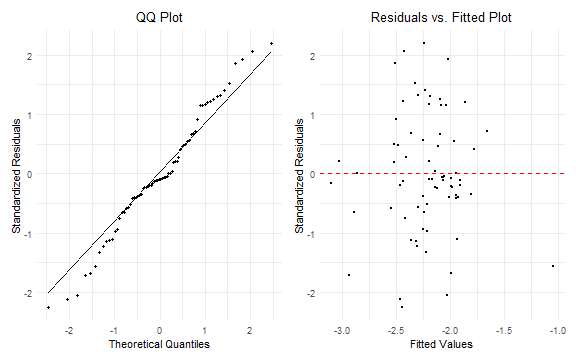## Wide-Form Data

Next, we will perform the same analysis as above, but will use the data in wide format.

We first clean the dataframe and convert it to wide format using functions from both the dplyr and tidyr packages

wi_w <- as.data.frame(wi_raw) %>%
dplyr::select(-moe) %>%
mutate(pov_prct = B17020_002/B17020_001,
feemp_prct = B23022_026/B23022_001,
year=id,
logpov = log(pov_prct),
logfeemp = log(pov_prct)) %>%
dplyr::select(-id, -(B17020_001:B23022_026), - geometry) %>%
relocate(year, .after=GEOID) %>%
gather(variable, value, -(GEOID:NAME)) %>%
unite(temp, variable, year) %>%

str(wi_w)

## 'data.frame':    72 obs. of  10 variables:
##  $GEOID : chr "55001" "55003" "55005" "55007" ... ##$ NAME           : chr  "Adams County, Wisconsin" "Ashland County, Wisconsin" "Barron County, Wisconsin" "Bayfield County, Wisconsin" ...
##  $feemp_prct_2017: num 0.443 0.495 0.486 0.492 0.497 ... ##$ feemp_prct_2018: num  0.442 0.491 0.491 0.491 0.498 ...
##  $logfeemp_2017 : num -2.04 -1.92 -2.16 -2.21 -2.18 ... ##$ logfeemp_2018  : num  -1.91 -1.94 -2.12 -2.16 -2.27 ...
##  $logpov_2017 : num -2.04 -1.92 -2.16 -2.21 -2.18 ... ##$ logpov_2018    : num  -1.91 -1.94 -2.12 -2.16 -2.27 ...
##  $pov_prct_2017 : num 0.13 0.146 0.115 0.11 0.113 ... ##$ pov_prct_2018  : num  0.148 0.143 0.12 0.115 0.103 ...

head(wi_w)

##   GEOID                       NAME feemp_prct_2017 feemp_prct_2018
## 1 55001    Adams County, Wisconsin       0.4432464       0.4415661
## 2 55003  Ashland County, Wisconsin       0.4953989       0.4910449
## 3 55005   Barron County, Wisconsin       0.4864993       0.4908756
## 4 55007 Bayfield County, Wisconsin       0.4923370       0.4906175
## 5 55009    Brown County, Wisconsin       0.4971482       0.4975893
## 6 55011  Buffalo County, Wisconsin       0.4800545       0.4845464
##   logfeemp_2017 logfeemp_2018 logpov_2017 logpov_2018 pov_prct_2017
## 1     -2.043496     -1.913802   -2.043496   -1.913802     0.1295749
## 2     -1.923672     -1.943329   -1.923672   -1.943329     0.1460696
## 3     -2.159050     -2.123234   -2.159050   -2.123234     0.1154347
## 4     -2.210303     -2.159529   -2.210303   -2.159529     0.1096674
## 5     -2.180429     -2.270736   -2.180429   -2.270736     0.1129930
## 6     -2.233846     -2.366587   -2.233846   -2.366587     0.1071156
##   pov_prct_2018
## 1    0.14751848
## 2    0.14322641
## 3    0.11964406
## 4    0.11537943
## 5    0.10323616
## 6    0.09380029

nrow(wi_w)

##  72


Notice how in this wide format, each variable is one of our poverty percent or female employment percent variables plus an associated year, and now we only have 72 rows in this wide format compared to 144 (72 counties $$\times$$ 2 time periods) rows.

### Exploratory (Spatial) Data Analysis

We will use the 2018 geometries for creating our neighborhood structure and list of spatial weights. To do this, we first create wi_2018geom, which is a simplified sf dataframe that only contains the GEOID variable and the associated county geometries.

wi_2018geom <- wi %>%
filter(year==2018) %>%
dplyr::select(GEOID, geometry)
str(wi_2018geom)

## Classes 'sf' and 'data.frame':   72 obs. of  2 variables:
##  $GEOID : chr "55001" "55003" "55005" "55007" ... ##$ geometry:sfc_MULTIPOLYGON of length 72; first list element: List of 1
##   ..$:List of 1 ## .. ..$ : num [1:311, 1:2] -90 -90 -90 -90 -90 ...
##   ..- attr(*, "class")= chr [1:3] "XY" "MULTIPOLYGON" "sfg"
##  - attr(*, "sf_column")= chr "geometry"
##  - attr(*, "agr")= Factor w/ 3 levels "constant","aggregate",..: NA
##   ..- attr(*, "names")= chr "GEOID"


Next, we will merge wi_2018geom (an sf dataframe that contains the county geometries) with wi_w (a regular dataframe) using the merge() function from the sp package:

#merge 2018 geometries back in
wi_w.sf <- merge(wi_2018geom, wi_w, by="GEOID")

nrow(wi_w.sf)

##  72

class(wi_w.sf)

##  "sf"         "data.frame"


Since our data is in wide format, we can create a similar map to the one created above in the long format, by creating 5 separate maps for each time period and then putting them together using the + operator from the patchwork R package. To create a title for the combined plot, we use plot_annotation() and to specify a single legend we use plot_layout(guides = 'collect'), both from the patchwork package.

p18 <- ggplot(wi_w.sf) +
geom_sf(aes(fill = logpov_2018)) +
scale_fill_viridis_c(limits = c(-3.2, -1)) +
coord_sf(datum = NA) +
theme_minimal() +
theme(plot.title=element_text(hjust = 0.5)) +
labs(title = "2018", fill="(Log) %Poverty")

p17 <- ggplot(wi_w.sf) +
geom_sf(aes(fill = logpov_2017)) +
scale_fill_viridis_c(limits = c(-3.2, -1)) +
coord_sf(datum = NA) +
theme_minimal() +
theme(plot.title=element_text(hjust = 0.5)) +
labs(title = "2017", fill="(Log) %Poverty")

#patchwork the plots together
patch1 <- p17 + p18
patch1 +
plot_annotation(title="County-Level (Log) Percent Poverty in Wisconsin Across Time") +
plot_layout(guides = "collect")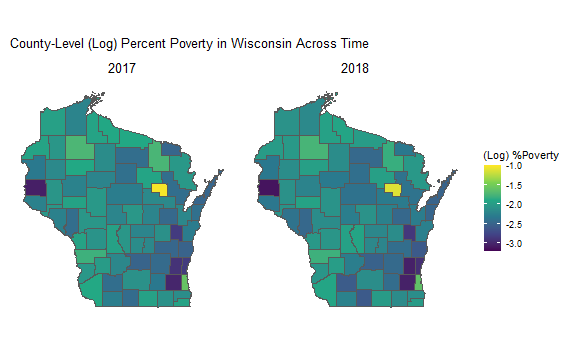### Create Neighbors

Creating the neighborhood structure and list of spatial weights is the same as above in long format.

row.names(wi_w.sf) <- wi_w.sf$NAME head(row.names(wi_w.sf))  ##  "Adams County, Wisconsin" "Ashland County, Wisconsin" ##  "Barron County, Wisconsin" "Bayfield County, Wisconsin" ##  "Brown County, Wisconsin" "Buffalo County, Wisconsin"  wi_nb_w <- poly2nb(wi_w.sf, row.names=row.names(wi_w.sf)) str(head(wi_nb_w))  ## List of 6 ##$ : int [1:6] 11 29 39 50 70 72
##  $: int [1:4] 4 26 51 58 ##$ : int [1:8] 7 9 17 49 55 56 58 66
##  $: int [1:4] 2 16 58 66 ##$ : int [1:6] 8 31 36 43 45 59
##  $: int [1:3] 18 47 62  listw_w <- nb2listw(wi_nb_w, style = "W") class(listw_w)  ##  "listw" "nb"  str(head(listw_w$weights))

## List of 6
##  $: num [1:6] 0.167 0.167 0.167 0.167 0.167 ... ##$ : num [1:4] 0.25 0.25 0.25 0.25
##  $: num [1:8] 0.125 0.125 0.125 0.125 0.125 0.125 0.125 0.125 ##$ : num [1:4] 0.25 0.25 0.25 0.25
##  $: num [1:6] 0.167 0.167 0.167 0.167 0.167 ... ##$ : num [1:3] 0.333 0.333 0.333

county_geoms <- st_geometry(wi_w.sf)
cntrd <- st_centroid(county_geoms)
coords <- st_coordinates(cntrd)

##           X        Y
## 1 -89.77039 43.96953
## 2 -90.67794 46.31608
## 3 -91.84831 45.42368
## 4 -91.20079 46.52376
## 5 -88.00373 44.45294
## 6 -91.75445 44.37983


To be sure that our neighbors look the same, we can again plot the county geometries, centroids, and neighborhood:

plot(county_geoms)### Using strm

The first argument is the model formula. Given that the data is now in wide format, we must manually enter the response and all of the explanatory variables and their lagged terms. The next argument is id, which we set to “NAME” as each observation is taken at the county-level. We then specify the name of the data set (data=wi_w.sf). We set the argument listw=listw_w to the row-standardized list of spatial weights. We set time=2 because there are 2 time periods in the dataset. We set wide=TRUE, thereby specifying that the data is in wide format.

formula2 <-as.formula(logpov_2018  ~feemp_prct_2017 + feemp_prct_2018 + logpov_2017)
out2 <- strm(formula2, id="NAME", data=wi_w.sf, listw= listw_w, time=2, wide=TRUE)

## The spatio-temporal regression model fitted:

## logpov_2018 ~ feemp_prct_2017 + feemp_prct_2018 + logpov_2017

summary(out2)

##
## Call:spatialreg::errorsarlm(formula = modframe, listw = listw)
##
## Residuals:
##        Min         1Q     Median         3Q        Max
## -0.1164060 -0.0271688 -0.0052208  0.0303804  0.1135471
##
## Type: error
## Coefficients: (asymptotic standard errors)
##                  Estimate Std. Error z value  Pr(>|z|)
## (Intercept)      0.490040   0.194409  2.5207 0.0117132
## feemp_prct_2017  8.009277   2.792433  2.8682 0.0041281
## feemp_prct_2018 -9.122176   2.765474 -3.2986 0.0009717
## logpov_2017      0.992349   0.018244 54.3924 < 2.2e-16
##
## Lambda: -0.35546, LR test value: 2.9886, p-value: 0.08385
## Asymptotic standard error: 0.18981
##     z-value: -1.8727, p-value: 0.061103
## Wald statistic: 3.5072, p-value: 0.061103
##
## Log likelihood: 110.748 for error model
## ML residual variance (sigma squared): 0.0026359, (sigma: 0.051341)
## Number of observations: 72
## Number of parameters estimated: 6
## AIC: -209.5, (AIC for lm: -208.51)


Compare the output from out$res from the long format dataframe and out2 from the wide format dataframe and see that they are the same! out_coefs <- c(as.vector(out$res$coefficients), out$res$lambda) out_ses <- c(as.vector(out$res$rest.se),out$res$lambda.se) out_names <- c(attributes(out$res$coefficients)[], "lambda") out2_coefs <- c(as.vector(out2$coefficients), out2$lambda) out2_ses <- c(as.vector(out2$rest.se), out2$lambda.se) out2_names <- c(attributes(out2$coefficients)[], "lambda")

out_table <- rbind.data.frame(cbind.data.frame(Term=out_names, Estimate=out_coefs, SE=out_ses, format=rep("Long format",length(out_names))),
cbind.data.frame(Term=out2_names, Estimate=out2_coefs, SE=out2_ses,format=rep("Wide format",length(out2_names))))

out_table %>%
gt(groupname_col = c("format")) %>%
tab_header(title="Comparison of Long and Wide format Output") %>%
fmt_number(columns = vars(Estimate, SE),  decimals = 2) %>%
cols_label(Term = md("**Term**"), Estimate=md("**Estimate**"), SE=md("**SE**"))


<!–html_preserve–>

Comparison of Long and Wide format Output
Term Estimate SE
Long format
(Intercept) 0.49 0.19
feemp_prct −9.12 2.77
feemp_prct.Tlag1 8.01 2.79
logpov.Tlag1 0.99 0.02
lambda −0.36 0.19
Wide format
(Intercept) 0.49 0.19
feemp_prct_2017 8.01 2.79
feemp_prct_2018 −9.12 2.77
logpov_2017 0.99 0.02
lambda −0.36 0.19
<!–/html_preserve–>

# Working with tidycensus

In this example, we have provided the data used. However the data can be downloaded directly using the tidycensus R package. The code to download the data directly is below. To use tidycensus, you will need to request an API key here.

#load tidycensus
library(tidycensus)
options(tigris_use_cache = TRUE)


## Downloading Census Data Using tidycensus

From the tidycensus package, we use the census_api_key() function and specify install=TRUE so that the API key is stored in the local R environment. To access the variables we want from the American Community Survey. It should be noted that using tidycensus, we can also access the 1990, 2000, and 2010 decennial US Census data as well.

census_api_key("YOUR API KEY HERE", install=TRUE)


(You may need to either restart your R session or run readRenviron("~/.Renviron") in your console to use the API key immediately.)

vars <- load_variables(2018, "acs5", cache=TRUE)
years <- lst(2017,2018)
multi_year <- map(years,~ get_acs(geography = "county",
variables = c("B17020_001","B17020_002","B23022_026","B23022_001"),
state = "WI",
year = .x,
geometry = TRUE)) %>%
map2(years, ~ mutate(.x, id = .y))

wi_raw <- reduce(multi_year, rbind)


For more examples of working with the tidycensus package, please see the vignettes for basic usage and working with spatial data. Full tidycensus support can be found here.